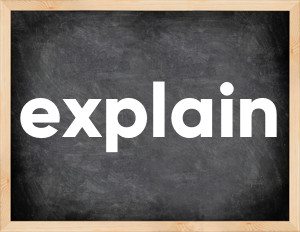# Explain past tenseThe English verb 'explain' is pronounced as [ɪkˈspleɪn].
Related to: regular verbs.
3 forms of verb explain: Infinitive (explain), Past Simple - (explained), Past Participle - (explained).

## Here are the past tense forms of the verb explain

👉 Forms of verb explain in future and past simple and past participle.
❓ What is the past tense of explain.

## Explain: Past, Present, and Participle Forms

Base Form Past Simple Past Participle
explain [ɪkˈspleɪn]

explained [ɪkˈspleɪnd]

explained [ɪkˈspleɪnd]

## What are the 2nd and 3rd forms of the verb explain?

🎓 What are the past simple, future simple, present perfect, past perfect, and future perfect forms of the base form (infinitive) 'explain'?

### Learn the three forms of the English verb 'explain'

• the first form (V1) is 'explain' used in present simple and future simple tenses.
• the second form (V2) is 'explained' used in past simple tense.
• the third form (V3) is 'explained' used in present perfect and past perfect tenses.

## What are the past tense and past participle of explain?

The past tense and past participle of explain are: explain in past simple is explained, and past participle is explained.

### What is the past tense of explain?

The past tense of the verb "explain" is "explained", and the past participle is "explained".

### Verb Tenses

Past simple — explain in past simple explained (V2).
Future simple — explain in future simple is explain (will + V1).
Present Perfect — explain in present perfect tense is explained (have/has + V3).
Past Perfect — explain in past perfect tense is explained (had + V3).

### explain regular or irregular verb?

👉 Is 'explain' a regular or irregular verb? The verb 'explain' is regular verb.

## Examples of Verb explain in Sentences

•   It also explained the main reason (Past Simple)
•   Her father has explained me that this thing was found in the crater (Past Simple)
•   How can you explain this smell? (Present Simple)
•   I thought I had explained it to her, but I was wrong (Past Perfect)
•   I'm tired of explaining you the most banal things (Present Continuous)
•   When I entered the room your son was explaining my daughter how to eat with chopsticks (Past Simple)
•   I don't think you can explain it, son (Present Simple)
•   I will explain you everything after I'm done fixing our washing machine (Future Simple)
•   Don't make me explain it to you (Present Simple)
•   I have been explaining him Present Perfect Continuous for half an hour (Present Perfect Continuous)

Along with explain, words are popular bury and undergo.

Verbs by letter: , , , , , , , , , , , , , , , , , , , , , , , , .